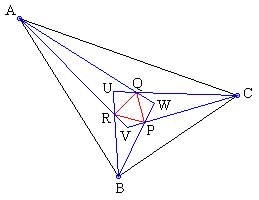### This applet requires Sun's Java VM 2 which your browser may perceive as a popup. Which it is not. If you want to see the applet work, visit Sun's website at https://www.java.com/en/download/index.jsp, download and install Java VM and enjoy the applet.

 What if applet does not run?

ExplanationCopyright © 1996-2018 Alexander Bogomolny

### Isogonal Concurrency

The applet suggests a theorem that was brought up in the discussion on Morley's theorem [Dale, p. 167].Assume at every vertex of ΔABC there are given a pair of isogonally conjugate lines: AV and AW, BU and BW, and CU and CV. Let P be the intersection of BV and CV, Q the intersection of AW and CU, and R the intersection of AW and BV. Then there are triplets of concurrent lines: PU, QV, RW AP, BQ, CR AU, BV, CW

On that occasion I was only concerned with a specific case of Hofstadter's concurrencies, where at every vertex the isogonal lines cut equal parts of the triangle ABC's angles. Quite recently, I discovered one of the concurrencies , viz., #2, in R. Honsberger's book, p. 153. The problem has been published by E. J. Hopkins in The Mathematical Gazette, 1950, 130-131. The problem there is more general and just repeats the above statement.

Honsberger gives a proof by L. C. Lyness, who derived the proportion

 BS/SC = (cot(b) - cot(B))/(cot(g) - cot(C)),

where B and C are angles of ΔABC, b and g are angles formed by isogonal lines with the corresponding sides, and S is the point of intersection of AP with BC. Taken cyclically for the other two sides, the proportions cancel out in the formulation of Ceva's theorem. So, by the latter, AP, BQ, CR are concurrent. The derivation, while elementary, is not obvious and requires additional construction.

### References

1. D. Gale, Tracking the Automatic Ant, Springer-Verlag, 1998
2. R. Honsberger, Mathematical Chestnuts from Around the World, MAA, 2001.Copyright © 1996-2018 Alexander Bogomolny

 65268501